Published © CC BY-NC

# A DIY Smart Insole to Check Your Pressure Distribution

The smart insole collects foot pressure data in real-time. Through Thinger.io the information is fed into a system that visualizes the data.

IntermediateFull instructions provided10 hours32,318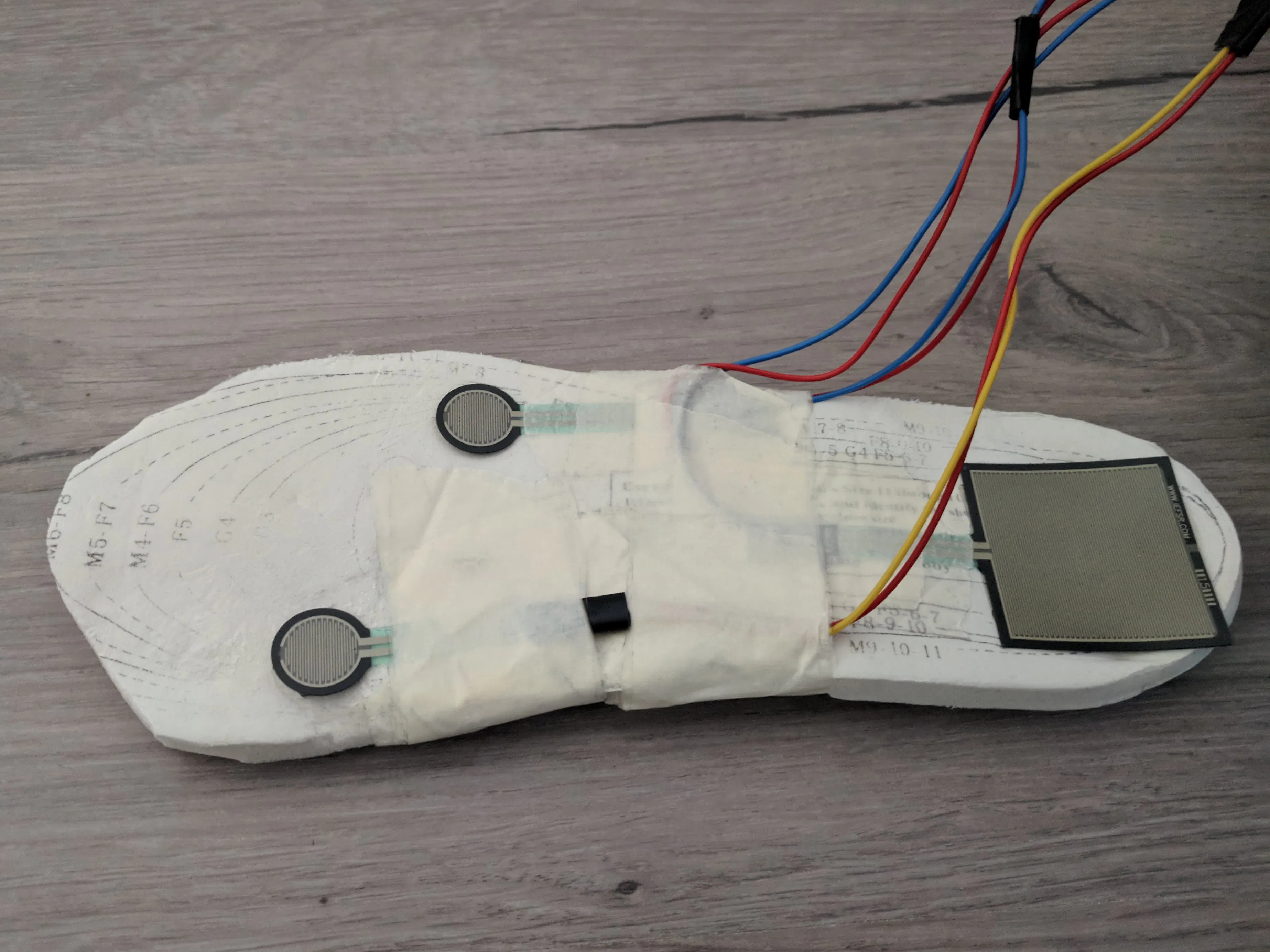## Things used in this project

### Hardware components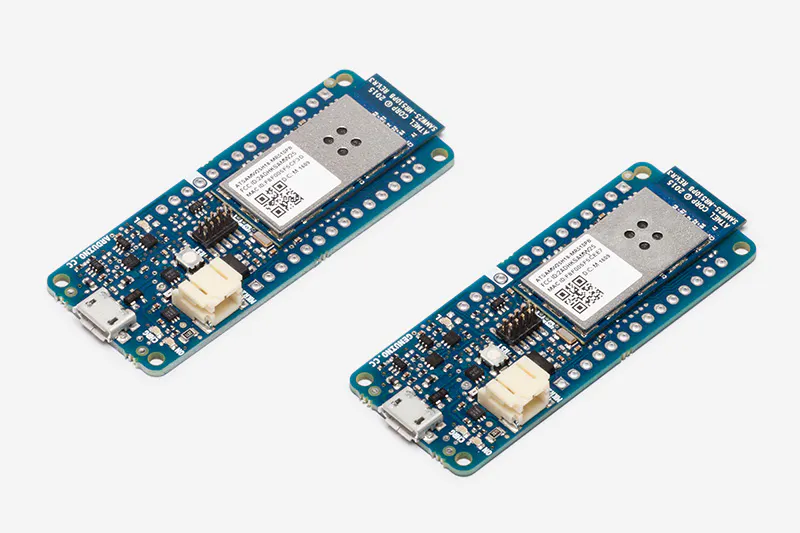Arduino MKR1000
×1
 Force Sensitive Resistor I've used Interlink model 402
×3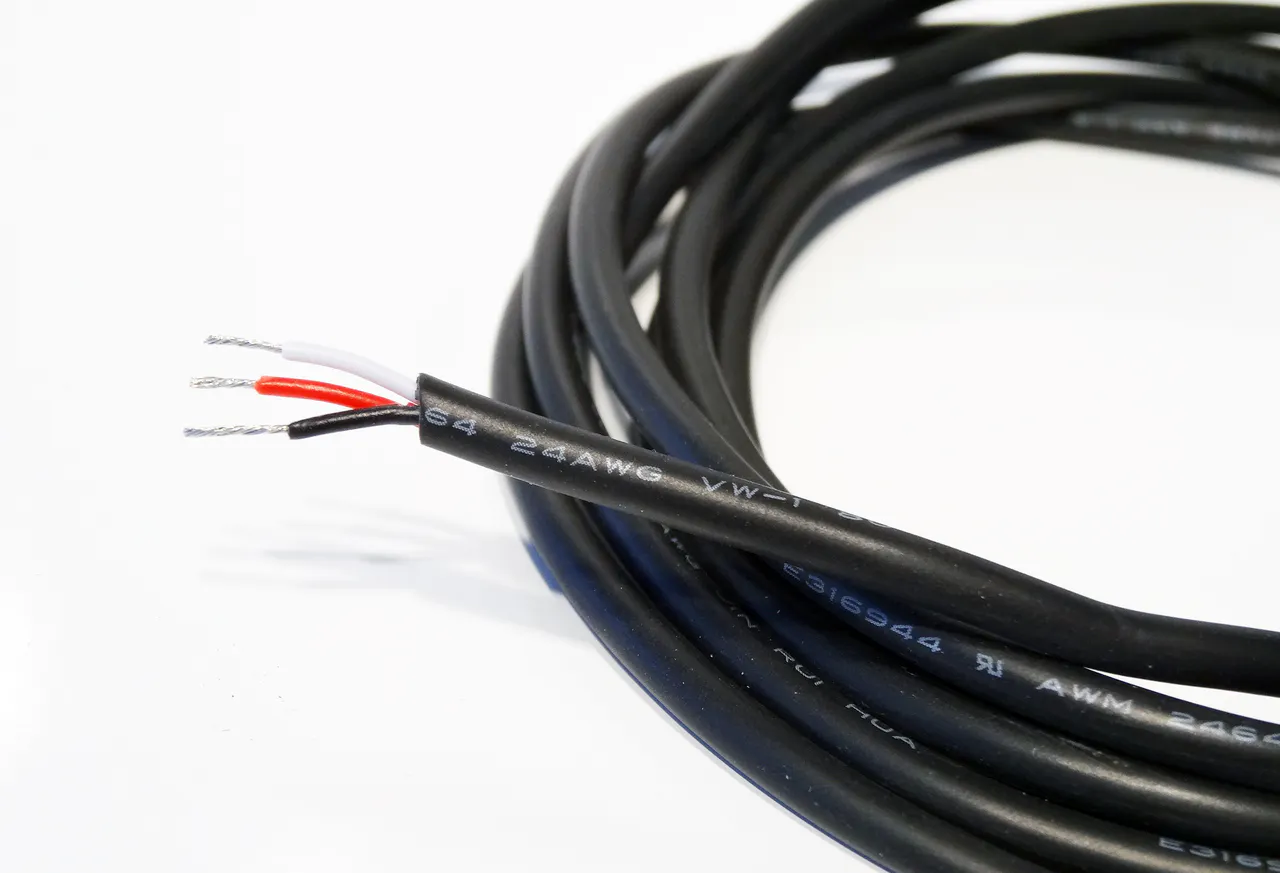OpenBuilds Wire Cable - By the Foot
×1Resistor 10k ohm
×3
 Power Bank
×1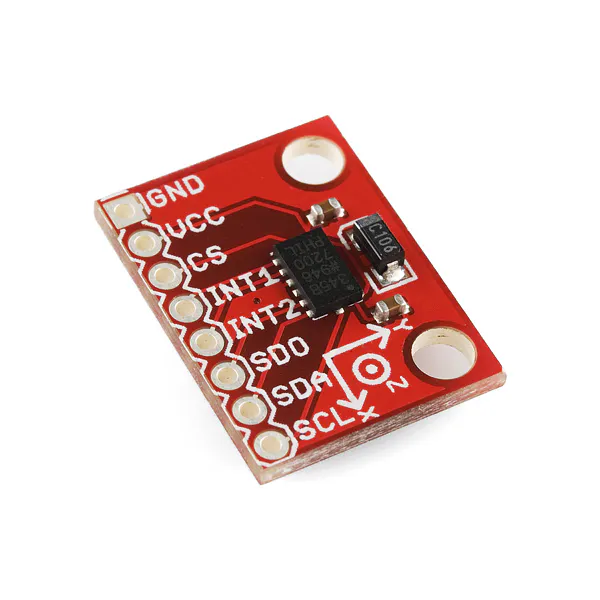SparkFun Triple Axis Accelerometer Breakout - ADXL345
×1

### Software apps and online servicesThinger.io PlatformArduino IDE

### Hand tools and fabrication machinesSoldering iron (generic) Don't forget to buy solder, heat shrink and other applicable accessoires if you don't have these

Read more

## Schematics

### Hardware

Three FSR's (with voltage dividers) and an accelerometer.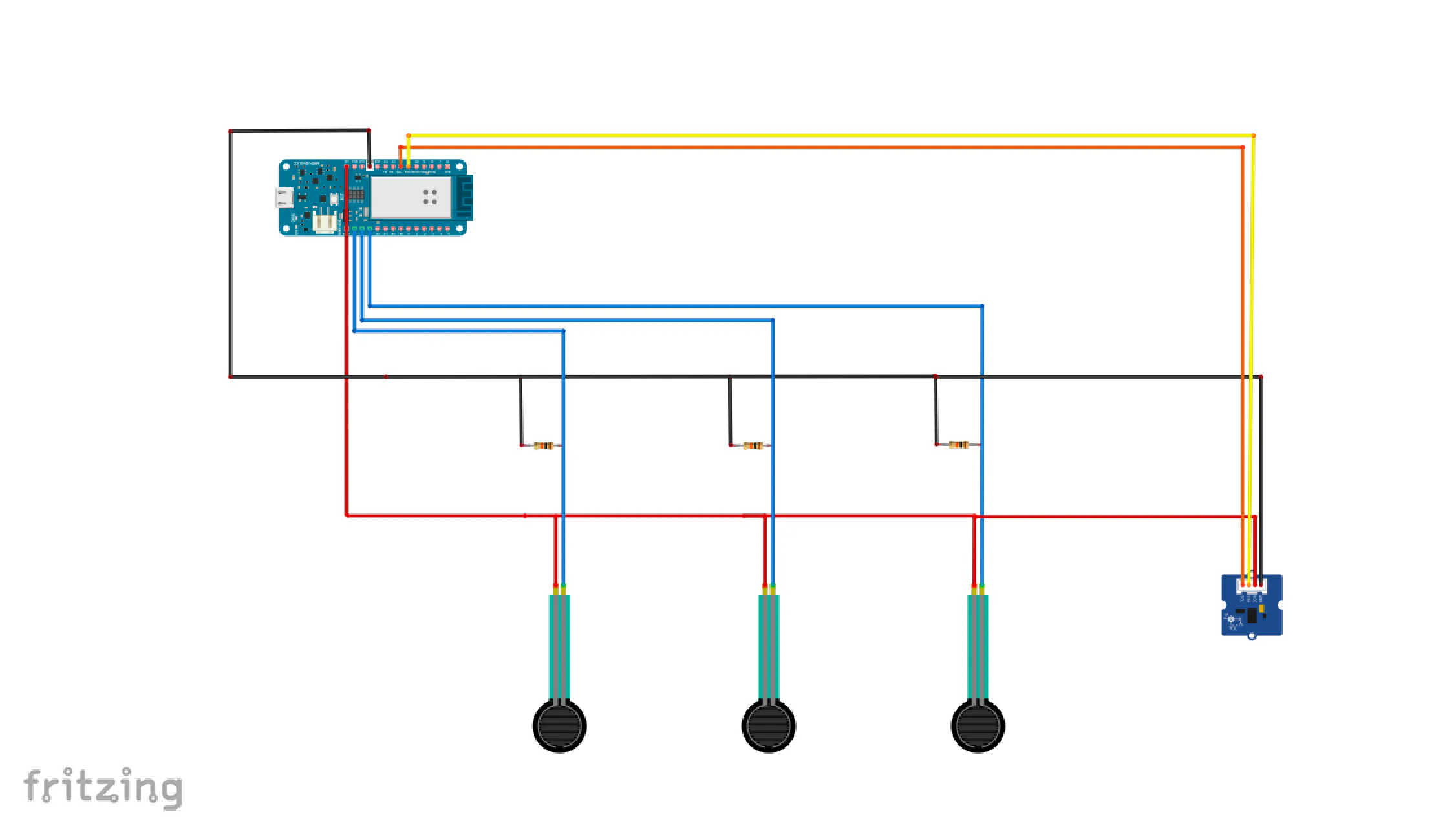## Code

### Smart-Insole-Tutorial

Arduino
Full code of this tutorial
```#define _DEBUG_  //Make sure this comes before any other includes or your board might crash

/*Please find the tutorial here: https://www.hackster.io/projects/a5ceae*/
#include <WiFi101.h> //Thinger
#include <ThingerWifi101.h> //Thinger
#include <Wire.h> //Accelerometer
#include <Adafruit_Sensor.h> //Accelerometer
#include <Adafruit_ADXL345_U.h> //Accelerometer

#define USERNAME "yourUsername"
#define DEVICE_ID "yourDevice"
#define DEVICE_CREDENTIAL "yourCredential"
#define SSID "yourSSID"
#define SSID_PASSWORD "yourSSIDPassword"

Adafruit_ADXL345_Unified accel = Adafruit_ADXL345_Unified(12345); //Accelerometer

int x = 0; //Reset to 0
int y = 0;
int z = 0;

//*FSR sensors*/
#define noFSRs 3 // Number of FSRs connected
#define FSR1 A1  //Analogue ports
#define FSR2 A2
#define FSR3 A3

float fsrVoltageArray;       // The analog reading converted and                                      //scaled to voltage as a floating point                                  //number
float fsrForceArray;         // The force in Newton
float fsrWeightInGramsArray; // Weight converted to grams

int   pinArray       = {FSR1, FSR2, FSR3};    // The pin ID for the                                                    //three devices
float forceMaxArray  = {100.0, 100.0, 100.0}; // Maximum forces                                                        //supported

float million = 1000000.0; // Unit for "1/micro
float conversionToKgrams = 1.0/9.80665;

long K       = 1000;
long R       = 10*K;    // R in K Ohm
long Vcc     = 5000;    // 5V=5000mV, 3.3V = 3300 mV
float voltageMax = 0.98 * Vcc; // Maximum voltage set to 95% of Vcc. Set                               //the force to the maximum beyond this                                 //value.

ThingerWifi101 thing(USERNAME, DEVICE_ID, DEVICE_CREDENTIAL);
//Call to set up WiFi function

void setup(void) {
Serial.begin(115200);
thing.add_wifi(SSID, SSID_PASSWORD);

if(!accel.begin()) {   //Initialise the sensor
Serial.println("No ADXL345 detected.");
} else {
accel.setRange(ADXL345_RANGE_16_G); //Range for this sensor

thing["accelerometer"] >> [](pson& out){
sensors_event_t event;
accel.getEvent(&event);
out["x"] = event.acceleration.x;
out["y"] = event.acceleration.y;
out["z"] = event.acceleration.z;
};
}

/*FSR sensors*/
thing["pressure"] >> [](pson & out) {
out["FSR1"] = analogRead(FSR1);
//    Serial.print("FSR1:");
//    Serial.println(analogRead(FSR1));
out["FSR2"] = analogRead(FSR2);
//    Serial.print("FSR2:");
//    Serial.println(analogRead(FSR2));

out["FSR3"] = analogRead(FSR3);
//    Serial.print("FSR3:");
//    Serial.println(analogRead(FSR3));
};

thing["voltage"] >> [](pson & out) {

for (int FSR = 0; FSR < noFSRs; FSR++) {

fsrVoltageArray[FSR] = 0.0; //Reset values upon entry
fsrForceArray[FSR]   = 0.0;

int fsrPin   = pinArray[FSR];
int fsrReading = analogRead(fsrPin);

fsrVoltageArray[FSR] = (float) map(fsrReading, 0, 1023, 0, 5000);
} //End of loop over FSR's

out["FSR1voltage"] = fsrVoltageArray;
out["FSR2voltage"] = fsrVoltageArray;
out["FSR3voltage"] = fsrVoltageArray;
};

thing["newton"] >> [](pson & out) {
for (int FSR = 0; FSR < noFSRs; FSR++) {

// The value of the force F as a function of the voltage V is           ///computed as: F(V) = (Fmax/Vmax) * V

float force_value = (forceMaxArray[FSR]/voltageMax) * fsrVoltageArray[FSR];

// Three situations are distinguished:
//
// 1. If V is too close to the maximum (as defined by voltageMax          // ), the force can
//    go to infinity. This is avoided by setting it the maximum          //value as soon as it is higher than our threshold voltageMax.
//
// 2. If the computed force F is too small, we set it to zero to          // avoid noise effects.
//
// 3. In all other cases, we take the logarithmic value to
//reduce the sloop and better distinguish small changes.

if ( fsrVoltageArray[FSR] < voltageMax ) {

// V is not too high in this branch

if ( force_value <= 1.00 ) {
fsrForceArray[FSR] = 0.0; // Force is too small, set it to                                        // zero
} else {
fsrForceArray[FSR] = log10(force_value); // Value is okay,                                                       //take the log of                                                       //this
}

} else {

// Cap the force if the voltage is too close to Vcc (for Vcc            //it would be infinity)

fsrForceArray[FSR] = log10(forceMaxArray[FSR]);

Serial.print("Cut off activated for FSR = "); Serial.println(FSR);
}

} // End of loop over FSRs
out["FSR1newton"] = fsrForceArray;
out["FSR2newton"] = fsrForceArray;
out["FSR3newton"] = fsrForceArray;
}; //End of thing

thing["weight"] >> [](pson & out) {

//Straightforward computation to convert the force in Newton to the weight in grams

for (int FSR = 0; FSR < noFSRs; FSR++) {
fsrWeightInGramsArray[FSR] = fsrForceArray[FSR] * conversionToKgrams * 1000.0;
}
out["FSR1weight"] = fsrWeightInGramsArray;
out["FSR2weight"] = fsrWeightInGramsArray;
out["FSR3weight"] = fsrWeightInGramsArray;
}; //End of thing
} //End of setup

void loop(void) {
thing.handle();
}
```

## Credits

### Juliette van der Pas

2 projects • 16 followers